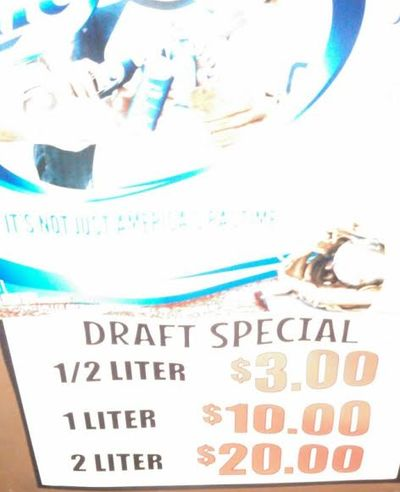# Happy hour prices Part-3(revised)

Algebra Level pendingA bar is offering greatly reduced prices on their draft beer during their happy hour. (See above image for menu prices)

If a group of friends decide that they want to drink a total of $25$ liters, how much would it cost them(minimum)?

Details and assumptions:

• The bartender doesn't know mathematics, and he always gives:

1) $\2$ extra for each $\dfrac 12$L drink you buy,

2) $\4$ extra for each $1$L drink you buy and,

3) $\11$ extra for each $2$L drink that you buy

• For example, if you buy a $1$L drink and pay him $\50$, he will give you $\44$ instead of $\40$.

• You can buy at most ten $\dfrac 12$L drink.

• You can buy drinks in natural numbers, i.e. you can't buy $12.5$ glasses of $2$L drink.

• You have just got $\50$ bills with you.

×